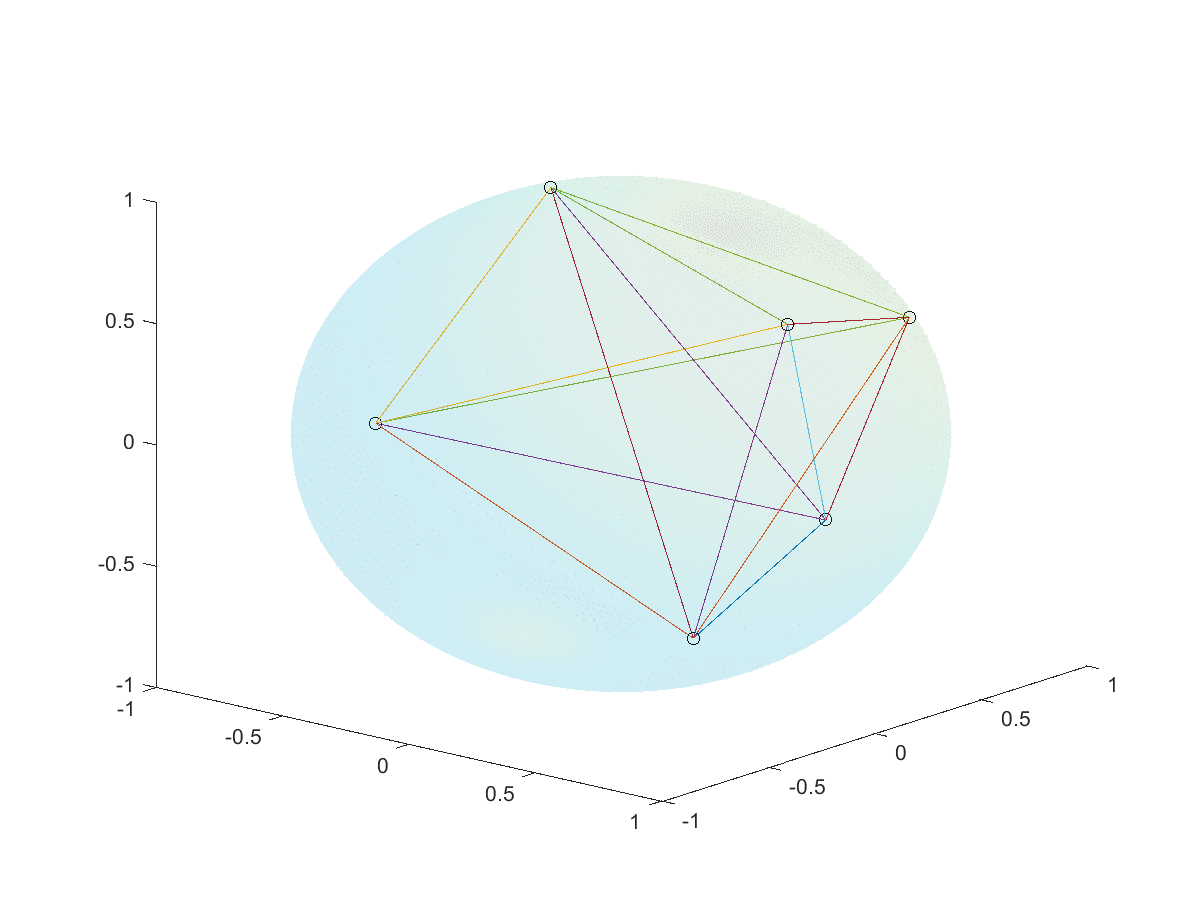The Python 🐍 problem-solving bootcamp 🚀 is starting soon. Join the second cohort now!

# Mathspp Blog

317 359,717 30,716
articles words lines of code
##### TIL #058 – do not cache generators

Today I learned not to cache generators.

##### Problem #041 – canyon crossing

It's night time and 4 friends need to cross a fragile bridge, but they only have one torch. What's the order in which they should cross?

##### Problem #030 - efficiency at the beach

You are sunbathing when you decide to go and talk to some friends under a nearby sun umbrella, but first you want to get your feet wet in the water. What is the most efficient way to do this?

##### Twitter proof: maximising the product with a fixed sum

Let's prove that if you want to maximise $$ab$$ with $$a + b$$ equal to a constant value $$k$$, then you want $$a = b = \frac{k}{2}$$.

##### A gradient descent algorithm to optimally distribute points over a sphere